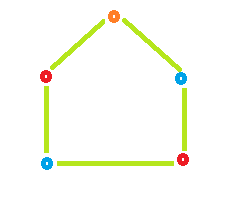# Coloring a Cycle Graph

• Difficulty Level : Basic
• Last Updated : 20 Aug, 2021

Cycle:- cycle is a path of edges and vertices wherein a vertex is reachable from itself. or in other words, it is a Closed walk.

Even Cycle:- In which Even number of vertices is present is known as Even Cycle.

Odd Cycle:- In which Odd number of Vertices is present is known as Odd Cycle.
Given the number of vertices in a Cyclic Graph. The task is to determine the Number of colors required to color the graph so that No two Adjacent vertices have the same color.

Approach:

If the no. of vertices is Even then it is Even Cycle and to color such graph we require 2 colors.
If the no. of vertices is Odd then it is Odd Cycle and to color such graph we require 3 colors.

Examples:

```Input : vertices = 3
Output : No. of colors require is: 3

Input : vertices = 4
Output : No. of colors require is: 2```

Example 1: Even Cycle: Number of vertices = 4Color required = 2Example 2: Odd Cycle: Number of vertices = 5Color required = 3## C++

 `// CPP program to find number of colors` `// required to color a cycle graph` `#include ` `using` `namespace` `std;`   `// Function that calculates Color` `// require to color a graph.` `int` `Color(``int` `vertices)` `{` `    ``int` `result = 0;`   `    ``// Check if number of vertices` `    ``// is odd or even.` `    ``// If number of vertices is even` `    ``// then color require is 2 otherwise 3` `    ``if` `(vertices % 2 == 0)` `        ``result = 2;` `    ``else` `        ``result = 3;`   `    ``return` `result;` `}`   `// Driver code` `int` `main()` `{` `    ``int` `vertices = 3;` `    ``cout << ``"No. of colors require is: "` `<< Color(vertices);` `    ``return` `0;` `}`

## Java

 `// Java program to find number of colors` `// required to color a cycle graph` `import` `java.io.*; `   `class` `GFG { ` `  `  `    ``// Function that calculates Color ` `    ``// require to color a graph.  ` `    ``static` `int` `Color(``int` `vertices) ` `    ``{ ` `        ``int` `result = ``0``; ` `  `  `        ``// Check if number of vertices ` `        ``// is odd or even. ` `        ``// If number of vertices is even ` `        ``// then color require is 2 otherwise 3 ` `        ``if` `(vertices % ``2` `== ``0``) ` `            ``result = ``2``; ` `        ``else` `            ``result = ``3``; ` `      `  `        ``return` `result; ` `    ``}  ` `      `  `    ``// Driver program to test above function ` `    ``public` `static` `void` `main (String[] args) ` `    ``{ ` `        ``int` `vertices = ``3``; ` `        `  `        ``System.out.println(``"No. of colors require is: "` `+ Color(vertices));` `          `  `    ``} ` `} `   `// this code is contributed by Naman_Garg`

## Python3

 `# Naive Python3 Program to ` `# find the number of colors` `# required to color a cycle graph  ` `  `  `# Function to find Color required.` `def` `Color(vertices):  ` `  `  `    ``result ``=` `0` `  `  `    ``# Check if number of vertices ` `    ``# is odd or even. ` `    ``# If number of vertices is even ` `    ``# then color require is 2 otherwise 3 ` `    ``if` `(vertices ``%` `2` `=``=` `0``):` `        ``result ``=` `2` `    ``else``:` `        ``result ``=` `3` `  `  `    ``return` `result` `  `  `# Driver Code ` `if` `__name__``=``=``'__main__'``:` `    ``vertices ``=` `3` `    ``print` `(``"No. of colors require is:"``,Color(vertices))`   `# this code is contributed by Naman_Garg`

## C#

 `// C# program to find number of colors` `// required to color a cycle graph` `using` `System; `   `class` `GFG` `{ `   `// Function that calculates Color ` `// require to color a graph. ` `static` `int` `Color(``int` `vertices) ` `{ ` `    ``int` `result = 0; `   `    ``// Check if number of vertices ` `    ``// is odd or even. ` `    ``// If number of vertices is even ` `    ``// then color require is 2 otherwise 3 ` `    ``if` `(vertices % 2 == 0) ` `        ``result = 2; ` `    ``else` `        ``result = 3; `   `    ``return` `result; ` `} `   `// Driver Code` `public` `static` `void` `Main () ` `{ ` `    ``int` `vertices = 3; ` `    `  `    ``Console.WriteLine(``"No. of colors required is: "` `+ ` `                                   ``Color(vertices));` `} ` `} `   `// This code is contributed by anuj_67`

## PHP

 ``

## Javascript

 ``

Output:

`No. of colors require is: 3`

My Personal Notes arrow_drop_up
Recommended Articles
Page :